# MP Board Class 10th Science Solutions Chapter 5 Periodic Classification of Elements

## MP Board Class 10th Science Solutions Chapter 5 Periodic Classification of Elements

### MP Board Class 10th Science Chapter 5 Intext Questions

Intext Questions Page No. 81

Question 1.
Did Dobereiner’s triads also exist in the columns of Newlands’ Octaves? Compare and find out.
In Newland’s Octaves, the properties of lithium and sodium were found to be the same. This arrangement is also found in Dobereiner triads.

Question 2.
What were the limitations of Dobereiner’s classification?
Dobereiner could identify only three ‘triads’ from the elements known at that time. Hence this system of classification into triads was not found to be useful.Question 3.
What were the limitations of Newlands’ Law of Octaves?

The Limitations of Newlands’ Law of Oclaves is as follows:

1. It was found that the Law of Octaves was applicable only upto calcium, as after calcium every eighth element did not possess properties similar to that of the first.
2. It was assumed by Newlands’ that only 56 elements existed in nature and no more elements would be discovered in the future. But later on, several new elements were discovered, whose properties did not fit into the Law of Octaves.
3. In order to fit elements into his Table, Newlands adjusted two elements in the same slot, but also put some unlike elements under the same note.

Intext Questions Page No. 85

Question 1.
Use Mendeleev’s Periodic Table to predict the formulae for the oxides of the following elements: K, C, Al, Si, Ba.

1. K is in I group. Its oxide is K2O
2. C, is in IV group, its oxide is CO2
3. Al, is in III group, its oxide is Al2O3
4. Si, is IV group, its oxide is SiO2
5. Ba, is in II group, its oxide is BaO

Question 2.
Besides gallium, which other elements have since been discovered that were left by Mendeleev in his Periodic Table? (any two)
Scandium and germanium.

Question 3.
What were the criteria used by Mendeleev in creating his Periodic Table?
Mendeleev used the relationship between the atomic masses of the elements and their physical and chemical properties. Among chemical properties, he examined the compound formed by elements with oxygen and hydrogen. He found that if the 63 elements known at that time were arranged in the increasing order of their atomic masses, the properties of elements and also formulae of their oxides and hydrides gradually changed from element to element and at a certain interval they suddenly started almost repealing relationship was expressed by Mendeleev’s periodic law. i,e the properties of examinants are the periodic functions of their atomic masses.

Question 4.
Why do you think the noble gases are placed in a separate group?
All noble gases are inert elements. Their properties are different from other elements and are the least reactive. Therefore, the noble gases are placed in a separate group.

Intext Questions Page No. 90

Question 1.
How could the Modern Periodic Table remove various anomalies of Mendeleev’s Periodic Table?

1. In the Modern periodic Table atomic number of an elements is a more fundamental property than its atomic mass.
2. The anomalous position of hydrogen can be discussed after we see what are the basis on which the position of an elements in the Modern Periodic Table depends.
3. The elements present in any one group have the same number of valence electrons.
4. Atoms of different elements with the same number of occupied shells are placed in the same period.
5. In the Modern Periodic Table, a zig-zag line separated metals from non-metals.Question 2.
Name two elements you would expect to show chemical reactions similar to magnesium. What is the basis for your choice?
Calcium (Ca) and Strontium (Sr) is expected to show chemical reactions similar to magnesium (Mg). This is because the number of valence electrons (2) is the same in all of these three elements and since chemical properties are due to valence electrons, they show the same chemical reactions.

Question 3.
Name:

1. Three elements that have a single electron in their outermost shells.
2. Two elements that have two electrons in their outermost shells.
3. Three elements with filled outermost shells.

1. Lithium (Li), Sodium (Na), and Potassium (K) has a single electron in their outermost shells.
2. Magnesium (Mg) and Calcium (Ca) have two electrons in their outermost shells.
3. Neon (Ne), Argon (Ar), and Xenon (Xe) have filled outermost shells.

Question 4.
Lithium, sodium, potassium are all metals that react with water to liberate hydrogen gas. Is there any similarity in the atoms of these elements?

Lithium, sodium and potassium – These three elements have one electron in their outermost orbit.

b) Helium is an unreactive gas and neon is a gas of extremely low reactivity. What, if anything, do their atoms have in common?
Both helium (He) and neon (Ne) have filled outermost shells. Helium has a duplet in its K shells, while neon has an octet in its L shells.

Question 5.
In the Modern Periodic Table, which are the metals among the first ten elements?
In the modem periodic table, Lithium and Beryllium are the metals among the first 10 elements.

Question 6.
By considering their position in the Periodic Table, which one of the following elements would you expect to have maximum metallic characteristic?
Ga Ge As Se Be
Since, ‘Be’ lies to the extreme left-hand side of the periodic table, ‘Be’ is the most metallic among the given elements.

### MP Board Class 10th Science Chapter 5 Ncert Textbook Exercises

Question 1.
Which of the following statements is not a correct statement about the trends when going from left to right across the periods of the periodic table.
(a) The elements become less metallic in nature.
(b) The number of valence electrons increases.
(c) The atoms lose their electrons more easily.
(d) The oxides become more acidic.
(c) The atoms lose their electrons more easily.

Question 2.
Element X forms a chloride with the formula XCl2, which is solid with a high melting point. X would most likely be in the same group of the Periodic table as:
(a) Na
(b) Mg
(c) Al
(d) Si
(b) Mg

Question 3.
Which element has:
(a) two shells, both of which are completely filled with electrons?
(b) Electronic configuration 2, 8, 2?
(c) a total of three shells, with four electrons in its valence shell?
(d) a total of two shells, with three electrons in its valence shell?
(e) twice as many electrons in its second shell as in its first shell?
(a) Neon
(b) Magnesium
(c) Silicon
(d) Boron
(e) Carbon

Question 4.
(a) What property do all elements in the same column of the Periodic table as Boron have in common?
(b) What property do all elements in the same column of the Periodic table as Fluorine have in common?
(a) Valency equal to 3.
(b) Valency equal to 1.Question 5.
An atom has electronic configuration 2, 8, 7.
(a) What is the atomic number of this element?
(b) To which of the following elements would it be chemically similar? (Atomic numbers are given in parentheses.)
N(7) F(9) P(15) Ar(18)
(a) The atomic number of this element is 17.
(b) It would be chemically similar to F(9) with configuration as 2, 7.

Question 6.
The position of three elements A, B and C in the Periodic table is shown below: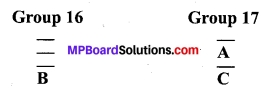(a) State whether A is a metal or non-metal.
(b) State whether C is more reactive or less reactive than A.
(c) Will C be larger or smaller in size than B?
(d) Which type of ion, cation or anion, will be formed by element A?
(a) A is a non-metal.
(b) C is less reactive than A because reactivity decreases down the group in halogens.
(c) C should be smaller in size than B as moving across a period, the nuclear charge increases and therefore, electrons come closer to the nucleus.
(d) A will form an anion as it will accept an electron to complete its octet.

Question 7.
Nitrogen (atomic number 7) and phosphorus (atomic number 15) belong to group 15 of the Periodic Table. Write the electronic configuration of these two elements. Which of these will be more electronegative? Why?
Nitrogen (7) : 2, 5
Phosphorus (15) : 2, 8, 5
Nitrogen is more electronegative because Metallic character decreases across a period and increased down a group.

Question 8.
How does the electronic configuration of an atom relate to its position in the Modern Periodic table?
In the modern periodic table, atoms with similar electronic configurations are placed in the same column. In a group, the number of valence electrons remains the same. Elements across a period show an increase in the number of valence electrons.

Question 9.
In the Modern Periodic table, calcium (atomic number 20) is surrounded by elements with atomic numbers 12, 19, 21, and 38. Which of these have physical and chemical properties resembling calcium?
The element with atomic number 12 has the same chemical properties as that of calcium. This is because both of them have same number of valence electrons (2).

Question 10.
Compare and contrast the arrangement of elements in Mendeleev’s Periodic table and the Modern Periodic table.

Mendeleev s Periodic table:

1. Elements are arranged in the increasing order of their atomic mass.
2. This table has 8 groups and 6 periods. And each group is subdivided as an A and B.
3. In this table, Hydrogen has no position.
4. No position for isotopes, because in Mendeleev period these are not discovered.

Modern Periodic table:

1. Elements are arranged in the increasing order of their atomic number.
2. It has 18 groups and 7 periods.
3. Inert gases are placed in separate groups.
4. In this table, a zigzag line separates Metals from Non-metals.

(or)

Mendeleev’s Periodic table vs Modern Periodic table:

1. Elements are arranged in the increasing order of their atomic masses, while in Modern Periodic table elements are arranged in the increasing order of their atomic numbers.
2. There are a total of 7 groups (columns) and 6 periods (rows) while in Mendeleev’s’ Periodic Table, there are a total of 18 groups (columns) and 7 periods (rows).
3. Elements having similar properties were placed directly under one another, while in Mendeleev’s’ Periodic Table elements having the same number of valence electrons are present in the same group.
4. In Mendeleev’s Periodic Table the position of hydrogen could not be explained, while in Modern Periodic table hydrogen is placed above alkali metals.
5. No distinguishing positions for metals and non-metals in Mendeleev’s Periodic Table while in Modern Periodic Table metals are present at the left-hand side of the periodic table whereas nonmetals are present at the right-hand side.

### MP Board Class 10th Science Chapter 5 Additional Questions

MP Board Class 10th Science Chapter 5 Multiple Choice Questions

Question 1.
Which of the following statements is correct about the trends when going down in a group of the periodic table?
(a) Elements become less electropositive in nature.
(b) Element oxides become more acidic.
(c) Valence electrons increases.
(d) Elements lose their electrons more easily.
(d) Elements lose their electrons more easily.

Question 2.
Element A forms a chloride with the formula ACl3, which is a stable compound. A would most likely be the same group of the Periodic Table as –
(a) Na
(b) Mg
(c) Al
(d) Si
(c) Al

Question 3.
Which of the following are coin metals?
(a) Ne, Ca, Na
(b) H2, N2, O2
(c) Li, Na, K
(d) Cu, Au, Ag
(d) Cu, Au, Ag

Question 4.
Who gave the triad arrangement of elements?
(a) Mendeleev
(b) Newlands
(c) Dalton
(d) Dobereiner
(d) Dobereiner

Question 5.
Newlands periodic table is based on the
(a) Atomic weight
(b) Atomic number
(d) Atomic volume
(a) Atomic weight

Question 6.
Which of the following is not gas in normal atmospheric condition?
(a) Helium (He)
(b) Argon (Ar)
(c) Bromine (Br)
(d) Chlorine (Cl)
(c) Bromine (Br)

Question 7.
While moving left to right across a period, the atomic radii –
(a) Remains the same
(b) Approaches zero
(c) Decreases
(d) Increases first then decreases
(c) Decreases

Question 8.
Which element is a metalloid?
(a) Carbon
(b) Nitrogen
(c) Oxygen
(d) Silicon
(d) SiliconQuestion 9.
Moseley’s periodic table is based on
(a) Atomic mass
(b) Mass number
(c) Atomic number
(d) Atomic volume
(c) Atomic number

Question 10.
Which of the following is a group of highly electronegative elements?
(a) Cl, Br, I
(b) S, Se, Te
(c) Na, K, Rb
(d) Ca, Sr, Ba
(a) Cl, Br, I

Question 11.
Which of the following elements is a non-metal?
(a) Aluminium
(b) Chlorine
(c) Sodium
(d) Silicon
(b) Chlorine

Question 12.
As we move down in a group in Modern Periodic Table, the size of elements generally
(a) increases
(b) decreases
(c) remain the same
(d) first, increase then decrease
(a) increases

Question 13.
As we move from top to bottom in a group in Modern Periodic Table, the electronegativity of elements
(a) Increases
(b) Decreases
(c) No change
(d) Not certain
(b) Decreases

Question 14.
Which group of elements is considered most electropositive?
(a) Group 1
(b) Group 2
(c) Group 17
(d) Group 18
(a) Group 1

Question 15.
Group 1 elements are also called as:
(a) Alkali metals
(b) Alkaline earth metals
(c) Halogens
(d) Noble gases
(a) Alkali metals

Question 16.
Group 17 elements are also called as:
(a) Alkali Metals
(b) Alkaline Earth Metals
(c) Halogens
(d) Noble Gases
(c) Halogens

Question 17.
How many elements were known when Mendeleev started his work?
(a) 100
(b) 215
(c) 65
(d) 80
(c) 65

Question 18.
Why Mendeleev left spaces in his Periodic Table?
(a) A mistake
(b) For future elements
(c) For Isotopes
(d) For Isobars
(b) For future elements

Question 19.
Why Lanthanoids and Actinoids are placed below in the Periodic Table?
(a) A mistake
(b) Better representation and view
(c) They were found very recently
(d) All of the above
(c) They were found very recently

Question 20.
A period may have elements with –
(a) Variable atomic sizes
(b) Variable atomic number
(c) Variable valency
(d) All of the above
(d) All of the above

Question 21.
Element A belongs to group 15. The formula of its hydride will:
(a) AH
(b) AH2
(c) AH3
(d) A3H
(c) AH3

Question 22.
An electropositive element, A with 2 valence electron will form which type of oxide?
(a) AO
(b) A2O
(c) AO2
(d) AO3
(a) AO

Question 23.
Most electronegative element in our Periodic Table:
(a) Iron
(b) Nitrogen
(c) Carbon
(d) Flourine
(d) Flourine

Question 24.
Which of the following elements where not among metals/elements named to fill the gap of Mendeleev’s Periodic Tablespaces?
(a) Cobalt
(b) Scandium
(c) Gallium
(d) Germanium
(a) Cobalt

Question 25.
In a group, all elements have similar ………..
(a) Electronic configuration
(b) Valence electron
(c) Electronegativity
(d) All of these
(b) Valence electronQuestion 26.
Which among the following is a noble gas?
(a) C
(b) N
(c) O
(d) Ne
(d) Ne

Question 27.
Which of the following elements has electronic configuration E = 2, 6?
(a) C
(b) N
(c) O
(d) Ne
(c) O

Question 28.
Which of the following elements is a metalloid?
(a) B
(b) Al
(c) S
(d) P
(a) B

Question 29.
Which of the following is not a halogen?
(a) Br
(b) I
(c) Te
(d) At
(c) Te

Question 30.
Which element has a total of two shells, with four valence electrons?
(a) C
(b) N
(c) Br
(d) Co
(a) C
(ii) Match column A’s description with column B’s Particulars.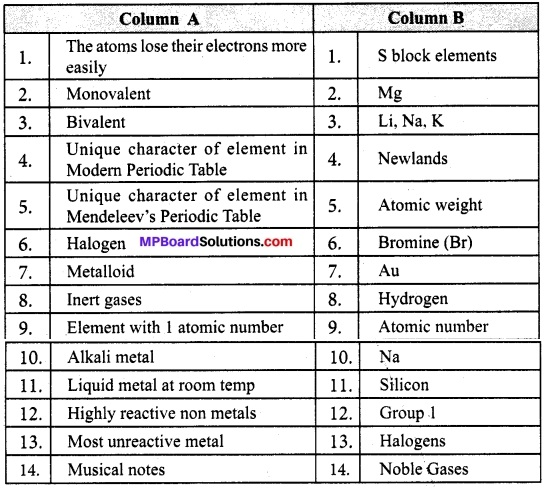1. → 12
2. → 3
3. → 2
4. → 4
5. → 5
6. → 13
7. → 11
8. → 14
9. → 10
10. → 10
11. → 6
12. → 1
13. → 7
14. → 8

MP Board Class 10th Science Chapter 5 Very Short Answer Type Questions

Question 1.
What would be the maximum number of electrons present in the outermost shell of atoms in the first period of Periodic Table?
Two

Question 2.
What is Solder?
It is an alloy of lead (Pb) and tin (Sn).

Question 3.
What is anode mud?
During electrolytic refining, the soluble impurities go into the solution, Whereas, the insoluble impurities settle down at the bottom of anode and are known as anode mud.

Question 4.
Which metal is used with iron oxide to join railway tracks or cracked machine parts?
Aluminium.

Question 5.
Give the thermit reaction.
Fe2O3(s) + 2Al(s) → 2 Fe(l) + Al2O3(s) + Heat

Question 6.
Roasting is used for the extraction of which ore?
Sulphide ore.

Question 7.
Name the metal lowest inactivity series (relative reactivities of metals).
Au or Gold.

Question 8.
Which gas is evolved when a metal reacts with nitric acid?
Hydrogen gas.

Question 9.
Name any two metal that does not react with water at all.
Lead, copper, gold, silver. (any two)

Question 10.
Complete the following reaction: Metal oxide + water →.
Metal hydroxide.

Question 11.
Which material is used to coat electric wires in homes?
PVC or Polyvinylchloride.Question 12.
Name any two metals that are poor conductors of heat.

MP Board Class 10th Science Chapter 5 Short Answer Type Questions

Question 1.
What are the limitations of the Modern Periodic Table?
The limitations of the Modern Periodic Table:
Position of hydrogen still dicey. It is not fixed till now. Position of lanthanides and actinides has not been given inside the main body of the Periodic Table. It does not reflect the exact distribution of electrons of some of the transition and inner transition elements.

Question 2.
Two elements X and Y have atomic numbers 12 and 16 respectively. Write the electronic configuration for these elements. To which period of the Modern Periodic Table do these two elements belong? What type of bond will be formed between them and why?
Electronic configuration of X (Z= 12): 2, 8,2
Electronic configuration of Y (Z = 16): 2, 8,6
Both these elements belong to the third period. An ionic bond is formed between X and Y due to the transfer of two electrons from X to Y.

Question 3.
The present classification of elements is based on which fundamental property of elements?
Atomic number.

Question 4.
Li, Na and K are the elements of a Dobereiner’s Triad. If the atomic mass of Li is 7 and that of K is 39, what would be the atomic mass of Na?
According to of Dobereiner’s law of triads, the atomic mass of the middle element, in this case, Na, should be the arithmetic mean of Li and K. Thus, Arithmetic mean of Li and K = (7 + 39)/2 = 23.

Question 5.
It states, “When elements are placed in order of the ascending order of atomic masses, groups of three elements having similar properties are obtained. The atomic mass of the middle element of the triad is equal to the mean of the atomic masses of the other two elements of the triad.”

Question 6.
Why did Dobereiner’s system of classification fail?
The major drawback of Döbereiner’s classification was that it was valid only for a few groups of elements known during that time. He was able to identify three triads only. Also, more accurate measurements of atomic masses showed that the mid element of the triad did not really have the mean value of the sum of the other two elements of the triad. For elements of very low mass or very high mass, the law did not hold good. For example, Fluorine (F), Chlorine (Cl), Bromine (Br). The atomic mass of Cl is not an arithmetic mean of atomic masses of F and Br.

Question 7.
Explain the position of metalloids in the Modern Periodic Table.
In the Modern Periodic Table, a zig-zag line separates metals from non-metals. The borderline elements – boron, silicon, germanium, arsenic, antimony, tellurium and polonium – are intermediate in properties and are called metalloids or semi-metals.

Question 8.
Why silicon is classified as metalloid?
Silicon is classified as a semi-metal or metalloid because it exhibits some properties of both metals and non-metals.

Question 9.
State Newlands law of octaves.
Elements are arranged in increasing order of their atomic masses such that the properties of the eighth element are the repetition of the properties of the first element (similar to eighth note in an octave of music).

Question 10.
X and Y are the two elements having similar properties which obey Newlands law of octaves. How many elements are there in between X and Y?
The law states there are eight elements in an octave (row). A number of elements between X and Y are six.

Question 11.
What are the drawbacks of Newlands law of octaves?
Following are the major drawbacks:

1. Worked well with lighter elements (upto calcium. After those elements in the eighth column did not possess properties similar to elements in the first column.
2. Newland assumed only 56 elements existed so far. Later, new elements were discovered which did not fit into octaves table.
3. Newland adjusted few elements in the same slot through their properties were quite different, e.g., Cobalt and nickel are in the same slot and these are placed in the same column as fluorine, chlorine and bromine which have very different properties than these elements. Iron, which resembles cobalt and nickel in properties, has been placed far away from these elements.

MP Board Class 10th Science Chapter 5 Long Answer Type Questions

Question 1.
What are the salient features of the Modern Periodic Table?
In a period of the Periodic Table, the number of valence electrons increases as the atomic number increases. As a result, elements change from metal to metalloid to nonmetal to a noble gas. Atomic size is a periodic property. As atomic number increases in a period, the atomic radius decreases. As atomic number increases in a group, atomic radius increases.

Positive ions have smaller atomic radii than the neutral atoms from which they derive. Negative ions have larger atomic radii than their neutral atoms. Positive ions in the same group increase in size down the group. In a group, each element has the same number of valence electrons. As a result, the elements in a group show similar chemical behaviour.

Metallic character decreases from left to right in a period because of the increase in the effective nuclear charge. Non-metallic character increase from left to right in a period because of the increase in effective nuclear charge. Non-metallic character decreases down the group because of increase in the size of the atom.Question 2.
What periodic trends do we observe in terms of atomic radii or atomic sizes in Modern Periodic table?
Following two trends are observed:
1. Within each column (group), atomic radius tends to increase from top to bottom. This trend results primarily from the increase in the number of the outer electrons. As we go down a column, the outer electrons have a greater probability of being farther from the nucleus, causing the atom to increase in size.

2. Within each row (period), the atomic radius tends to decrease from left to right. The major factor influencing this trend is the increase in the nuclear charge as we move across a row. The increasing effective nuclear charge steadily draws the valence electrons closer to the nucleus, causing the atomic radius to decrease.

Question 3.
An element A with atomic number 19 combines separately with NO3and (SO4)2,(PO4)3radicals:
(a) Give the electronic configuration of element A.
(b) Write the formulae of the three compounds so formed.
(c) To which group of the periodic table does the element ‘R’ belong?
(d) Does it form covalent or ionic compound? Why?
(a) Electronic configuration of A: 2,8, 8, 1.
(b) Compounds formed are A(NO3), A2(SO4) and K3(PO4).
(c) A has one valence electron and hence, it belongs to the first group.
(d) It forms the ionic compound.

Question 4.
Describe types of periods, blocks and trends of periodic properties along periods associated with Modern Periodic Table.
Periods:
First period (Atomic number 1 and 2): This is the shortest period. It contains only two elements (hydrogen and helium).

Second Period: (Atomic number 3 to 10): It contains eight elements (lithium to neon).

Third period (Atomic number 11 to 18): It contains eight elements (sodium to argon).

Fourth period (Atomic number 19 to 36): Row contains eighteen elements (potassium to krypton). i.e., 8 normal elements and 10 transition elements.

5th period (Atomic number 37 to 54): Contains 18 elements (rubidium to xenon) includes 8 normal elements and 10 transition elements.

Sixth period (Atomic number 55 to 86): The longest period. It contains 32 elements (caesium to radon) has 8 normal elements, 10 transition elements and 14 inner transition elements (lanthanides).

7th period (Atomic number 87 to 118): As like the sixth period, this period also can accommodate 32 elements. Till now 26 elements have been authenticated by IUPAC.

Blocks in Periodic Table:
The periodic table includes “blocks” defined in terms of which type of orbital is being filled via the Aufbau principle. This gives us the s-block, p-block, d-block, and f-block.

Blocks:
The s-, p-, d-, and f-blocks contain elements with outer electrons in the same type of orbital. Another key link between electron arrangement and position in the periodic table is that elements in any one main group have the same number of electrons in their highest energy level. The number of elements discovered so far is 118. The last element authenticated by IUPAC is Cn112 (Copernicium).

Properties of Periods: As you proceed to the left in a period or as you proceed down within a group:

1. The metallic strength increases (Non-Metallic Strength decreases).
3. The ionization potential decreases.
4. The electron affinity decreases.
5. The electronegativity decreases.

### MP Board Class 10th Science Chapter 5 Textbook Activities

Class 10 Science Activity 5.1 Page No. 84

1. Looking at its resemblance to alkali metals and the halogen family, try to assign hydrogen a correct position in Mendeleev’s Period Table.
2. To which group and period should hydrogen be assigned?

Observations:

1. No position can be fixed for hydrogen in the Mendeleev’s Periodic Table.
2. Properties of hydrogen fit with alkali metal as it combines with halogens, oxygen and sulphur to form compounds.
3. Properties of hydrogen also fit or are similar to halogen as it exists in the form of diatomic molecules and combines with metals and non-metals forming covalent compounds.

Class 10 Science Activity 5.2 Page No. 85

1. Consider the isotopes of chlorine, Cl-35 and CI-37.
2. Would you place them in different slots because their atomic masses are different?
3. Or would you place them in the same position because their chemical properties are the same?

Observations:
Two isotopes of chlorine are Cl-35 and Cl-37. Both isotopes have the same chemical properties and hence, both isotopes should be placed in the same position.

Class 10 Science Activity 5.3 Page No. 85

1. How were the positions of cobalt and nickel resolved in the Modern Periodic Table?
2. How were the positions of isotopes of various elements decided in the Modern Periodic Table?
3. Is it possible to have an element with atomic number 1, 5 placed between hydrogen and helium?
4. Where do you think should hydrogen be placed in the Modern Periodic Table?

Observations:
The position of Cobalt and Nickel were decided by placing them in the increasing order of atomic number in the Modern Periodic Table. Since isotopes are elements with the similar atomic number they are placed in the same position as its basic elements in the modern periodic table.

Class 10 Science Activity 5.4 Page No. 87

1. Look at group 1 of the Modern Periodic Table, and name the elements present in it. Write down the electronic configuration of the first three elements of group 1.
2. What similarity do you find in their electronic configurations?
3. How many valence electrons are present in these three elements?

Observations:
Elements present in Group 1 are: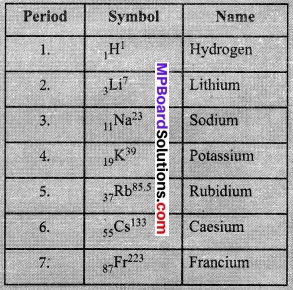Electronic configuration of the first three elements of Group I are as below: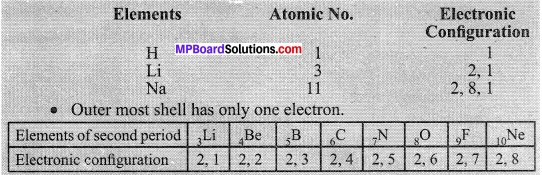Class 10 Science Activity 5.6 Page No. 87

1. If you look at the Modern Periodic Table, you will find that the elements Li, Be, B, C, N, O, F, and Ne are present in the second period. Write down their electronic configurations.
2. Do these elements also contain the same number of valence electrons?
3. Do they contain the same number of shells?

Observations:
No, these elements contain variable valence electrons as they belong to different groups: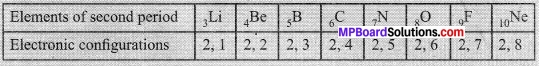Class 10 Science Activity 5.6 Page No. 88

1. How do you calculate the valency of an element from its electronic configuration?
2. What is the valency of magnesium with atomic number 12 and sulphur with atomic number 16?
3. Similarly, find out the valencies of the first twenty elements.
4. How does the valency vary in a period on going from left to right?
5. How does the valency vary in going down a group?

Observations:

1. Valency of an element can be calculated by the numbers of valence electron present.
2. Valency of Magnesium: 2
3. Valency of Sulphur: 2 Variation of valency while moving left to right in a period.
1 → 2 → 3 → 4 → 3 → 2 → 1 → 0
4. Variation of valency while going down in a group does not change.

Class 10 Science Activity 5.7 Page No. 88

1. Atomic radii of the elements of the second period are given below: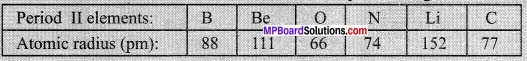2. Arrange them in decreasing order of their atomic radii.
3. Are the elements now arranged in the pattern of a period in the Periodic Table?
4. Which elements have the largest and the smallest atoms?
5. How does the atomic radius change as you go from left to right in a period?

Observations:
Decreasing order of atomic radii of following elements:

1. O < N < C < B < Be < Li
2. No in pattern.
3. Oxygen is smallest as per given data while Li is largest.
4. Atomic radius reduces while moving right in a group a, nuclear charge increase.

Class 10 Science Activity 5.8 Page No. 89

1. Study the variation in the atomic radii of first group elements given below and arrange them in increasing order.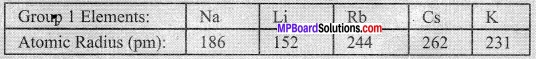2. Name the elements which have the smallest and the largest atoms.
3. How does the atomic size vary as you go down a group?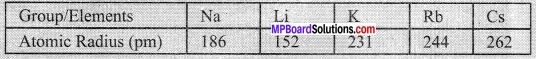1. Sodium (Na) has the smallest atom and calcium (Ca) has the largest atom.
2. Atomic size increases as we go down a group.

Class 10 Science Activity 5.9 Page No. 89

1. Examine elements of the third period and classify them as metals and non-metals.
2. On which side of the Periodic Table do you find the metals?
3. On which side of the Periodic Table do you find the non-metals?

Observations:
Elements of the third period are: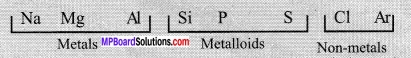Class 10 Science Activity 5.10 Page No. 89

1. How do you think the tendency to lose electrons change in a group?
2. How will this tendency change in a period?

Observations:
Metallic property reduces while moving right in a period.

Class 10 Science Activity 5.11 Page No. 90

1. How would the tendency to gain electrons change as you go from left to right across a period? How
2. would the tendency to gain electrons change as you go down a group?

Observations:

1. The electrons increases as we go left to right in a period up to 17th group. It decreases in the 18th
2. group. The tendency of gaining the electrons decreases as we go down a group.